# SobolIndicesAlgorithm¶

class SobolIndicesAlgorithm(*args)

Sensitivity analysis.

Notes

This method analyzes the influence of each component of an input random vector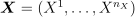on an output random vector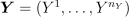by computing Sobol’ indices (see [sobol1993]). It computes, for every output random variable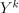(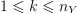), the part of its variance due to each input component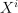(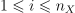) of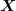.

Let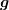be the physical model such as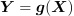. Let us first consider the case where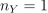. In that case, we denote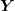by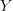. Let us write the variance ofas a function of. For any subset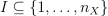, define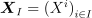.

Using the Hoeffding decomposition, we get: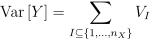where: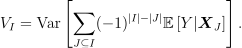For the sake of conciseness, for any integers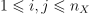, define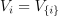and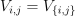. We have: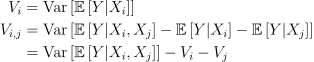for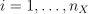.

First and second order Sobol’ indices are defined as follows:for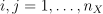.

The first order Sobol’ index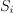measures the part of the variance ofexplained by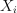alone. The second order Sobol’ index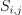measures the part of the variance ofexplained by the interaction ofand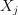.

For any, let us define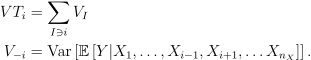Total order Sobol’ indices are defined as follows: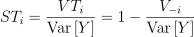for.

The total order Sobol’ index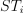quantifies the part of the variance ofthat is due to the effect ofand its interactions with all the other input variables. It can also be viewed as the part of the variance ofthat cannot be explained without.

In practice, to estimate these quantities, Sobol’ proposes to use numerical methods that rely on two independent realizations of the random vector. Let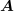and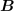be two independent samples (of size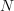) of:Each line is a realization of the random vector.

We are now going to mix these two samples to get an estimate of the sensitivity indices.For the sake of stability, computations will be performed with centered output. Let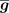be the mean of the combined samples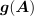and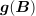. Let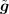be the empirically centered function defined, for any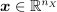, by: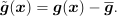To estimate the total variance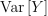, we use the computeVariance() method of the Sample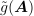.

Several estimators of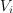,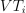and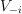are provided by the SobolIndicesAlgorithm implementations:

Specific formulas for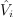,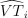and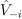are given in the corresponding documentation pages.

The estimator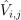of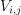is the same for all these classes: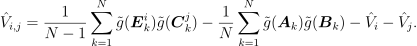Notice that the value of the second order conditional variance depends on the estimatorsand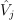which are method-dependent. This implies that the value of the second order indices may depend on the specific Sobol’ estimator we use.

For multivariate outputs i.e. when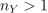, we compute the Sobol’ indices with respect to each output variable. In this case, the methods getFirstOrderIndices() and getTotalOrderIndices() return the Sobol’ indices of the first output, but the index of the output can be specified as input argument. Moreover, the indices can be aggregated [gamboa2013]. Let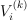be the (first order) variance of the conditional expectation of the k-th output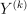: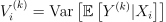forand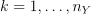. Similarily, let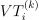be the total variance of the conditional expectation offorand.

The indices can be aggregated with the following formulas:for.

Aggregated indices can be retrieved with the getAggregatedFirstOrderIndices() and getAggregatedTotalOrderIndices() methods.

Notice that the distribution of the estimators of the first and total order indices can be estimated thanks to the getFirstOrderIndicesDistribution() and getTotalOrderIndicesDistribution() methods. This is done either through bootstrapping or using an asymptotic estimator. The ResourceMap key SobolIndicesAlgorithm-DefaultUseAsymptoticDistribution stores a boolean that decides the default behavior, but it can be overridden by the method setUseAsymptoticDistribution().

Corresponding confidence intervals are provided by the methods getFirstOrderIndicesInterval() and getTotalOrderIndicesInterval(). Their confidence level can be adjusted with setConfidenceLevel(). The default confidence level is stored in the ResourceMap and can be accessed with the SobolIndicesAlgorithm-DefaultConfidenceLevel key.

Indices estimates can be slightly outside of [0,1] if the estimator has not converged. For the same reason some first order indices estimates can be greater than the corresponding total order indices estimates.

The asymptotic estimator of the distribution requires an asymptotic estimate of its variance, which is computed using the [janon2014] delta method, as expained in the technical report [pmfre01116].

Methods

 Draw the correlation coefficients. Draw the importance factors. DrawSobolIndices(*args) Draw the Sobol' indices. draw(*args) Draw sensitivity indices. Get the evaluation of aggregated first order Sobol indices. Get the evaluation of aggregated total order Sobol indices. Get the number of bootstrap sampling size. Accessor to the object's name. Get the confidence interval level for confidence intervals. getFirstOrderIndices([marginalIndex]) Get first order Sobol indices. Get the distribution of the aggregated first order Sobol indices. Get interval for the aggregated first order Sobol indices. Accessor to the object's id. Accessor to the underlying implementation. Accessor to the object's name. getSecondOrderIndices([marginalIndex]) Get second order Sobol indices. getTotalOrderIndices([marginalIndex]) Get total order Sobol indices. Get the distribution of the aggregated total order Sobol indices. Get interval for the aggregated total order Sobol indices. Select asymptotic or bootstrap confidence intervals. setBootstrapSize(bootstrapSize) Set the number of bootstrap sampling size. setConfidenceLevel(confidenceLevel) Set the confidence interval level for confidence intervals. setDesign(inputDesign, outputDesign, size) Sample accessor. setName(name) Accessor to the object's name. Select asymptotic or bootstrap confidence intervals.
__init__(*args)
static DrawCorrelationCoefficients(*args)
Draw the correlation coefficients.

As correlation coefficients are considered, values might be positive or negative.

Available usages:

DrawCorrelationCoefficients(correlationCoefficients, title=’Correlation coefficients’)

DrawCorrelationCoefficients(values, names, title=’Correlation coefficients’)

Parameters:
correlationCoefficientsPointWithDescription

Sequence containing the correlation coefficients with a description for each component. The descriptions are used to build labels for the created graph. If they are not mentioned, default labels will be used.

valuessequence of float

Correlation coefficients.

namessequence of str

Variables’ names used to build labels for the created the graph.

titlestr

Title of the graph.

Returns:
graphGraph

A graph containing a Cloud and a Text of the correlation coefficients.

static DrawImportanceFactors(*args)

Draw the importance factors.

Available usages:

DrawImportanceFactors(importanceFactors, title=’Importance Factors’)

DrawImportanceFactors(values, names, title=’Importance Factors’)

Parameters:
importanceFactorsPointWithDescription

Sequence containing the importance factors with a description for each component. The descriptions are used to build labels for the created Pie. If they are not mentioned, default labels will be used.

valuessequence of float

Importance factors.

namessequence of str

Variables’ names used to build labels for the created Pie.

titlestr

Title of the graph.

Returns:
graphGraph

A graph containing a Pie of the importance factors of the variables.

static DrawSobolIndices(*args)

Draw the Sobol’ indices.

Parameters:
inputDescriptionsequence of str

Variable names

firstOrderIndicessequence of float

First order indices values

totalOrderIndicessequence of float

Total order indices values

fo_ciInterval, optional

First order indices confidence interval

to_ciInterval, optional

Total order indices confidence interval

Returns:
graphGraph

For each variable, draws first and total indices

draw(*args)

Draw sensitivity indices.

Usage:

draw()

draw(marginalIndex)

With the first usage, draw the aggregated first and total order indices. With the second usage, draw the first and total order indices of a specific marginal in case of vectorial output

Parameters:
marginalIndex: int

marginal of interest (case of second usage)

Returns:
graphGraph

A graph containing the aggregated first and total order indices.

Notes

If number of bootstrap sampling is not 0, and confidence level associated > 0, the graph includes confidence interval plots in the first usage.

getAggregatedFirstOrderIndices()

Get the evaluation of aggregated first order Sobol indices.

Returns:
indicesPoint

Sequence containing aggregated first order Sobol indices.

getAggregatedTotalOrderIndices()

Get the evaluation of aggregated total order Sobol indices.

Returns:
indicesPoint

Sequence containing aggregated total order Sobol indices.

getBootstrapSize()

Get the number of bootstrap sampling size.

Returns:
bootstrapSizeint

Number of bootstrap sampling

getClassName()

Accessor to the object’s name.

Returns:
class_namestr

The object class name (object.__class__.__name__).

getConfidenceLevel()

Get the confidence interval level for confidence intervals.

Returns:
confidenceLevelfloat

Confidence level for confidence intervals

getFirstOrderIndices(marginalIndex=0)

Get first order Sobol indices.

Parameters:
iint, optional

Index of the output marginal of the function, equal to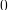by default.

Returns:
indicesPoint

Sequence containing first order Sobol indices.

getFirstOrderIndicesDistribution()

Get the distribution of the aggregated first order Sobol indices.

Returns:
distributionDistribution

Distribution for first order Sobol indices for each component.

getFirstOrderIndicesInterval()

Get interval for the aggregated first order Sobol indices.

Returns:
intervalInterval

Interval for first order Sobol indices for each component. Computed marginal by marginal (not from the joint distribution).

getId()

Accessor to the object’s id.

Returns:
idint

Internal unique identifier.

getImplementation()

Accessor to the underlying implementation.

Returns:
implImplementation

A copy of the underlying implementation object.

getName()

Accessor to the object’s name.

Returns:
namestr

The name of the object.

getSecondOrderIndices(marginalIndex=0)

Get second order Sobol indices.

Parameters:
iint, optional

Index of the marginal of the function, equals toby default.

Returns:
indicesSymmetricMatrix

Tensor containing second order Sobol indices.

getTotalOrderIndices(marginalIndex=0)

Get total order Sobol indices.

Parameters:
iint, optional

Index of the output marginal of the function, equal toby default.

Returns:
indicesPoint

Sequence containing total order Sobol indices.

getTotalOrderIndicesDistribution()

Get the distribution of the aggregated total order Sobol indices.

Returns:
distributionDistribution

Distribution for total order Sobol indices for each component.

getTotalOrderIndicesInterval()

Get interval for the aggregated total order Sobol indices.

Returns:
intervalInterval

Interval for total order Sobol indices for each component. Computed marginal by marginal (not from the joint distribution).

getUseAsymptoticDistribution()

Select asymptotic or bootstrap confidence intervals.

Returns:
useAsymptoticDistributionbool

Whether to use bootstrap or asymptotic intervals

setBootstrapSize(bootstrapSize)

Set the number of bootstrap sampling size.

Default value is 0.

Parameters:
bootstrapSizeint

Number of bootstrap sampling

setConfidenceLevel(confidenceLevel)

Set the confidence interval level for confidence intervals.

Parameters:
confidenceLevelfloat

Confidence level for confidence intervals

setDesign(inputDesign, outputDesign, size)

Sample accessor.

Allows one to estimate indices from a predefined Sobol design.

Parameters:
inputDesignSample

Design for the evaluation of sensitivity indices, obtained thanks to the SobolIndicesAlgorithmImplementation.Generate method

outputDesignSample

Design for the evaluation of sensitivity indices, obtained as the evaluation of a Function (model) on the previous inputDesign

Nint

Base size of the Sobol design

setName(name)

Accessor to the object’s name.

Parameters:
namestr

The name of the object.

setUseAsymptoticDistribution(useAsymptoticDistribution)

Select asymptotic or bootstrap confidence intervals.

Default value is set by the SobolIndicesAlgorithm-DefaultUseAsymptoticDistribution key.

Parameters:
useAsymptoticDistributionbool

Whether to use bootstrap or asymptotic intervals